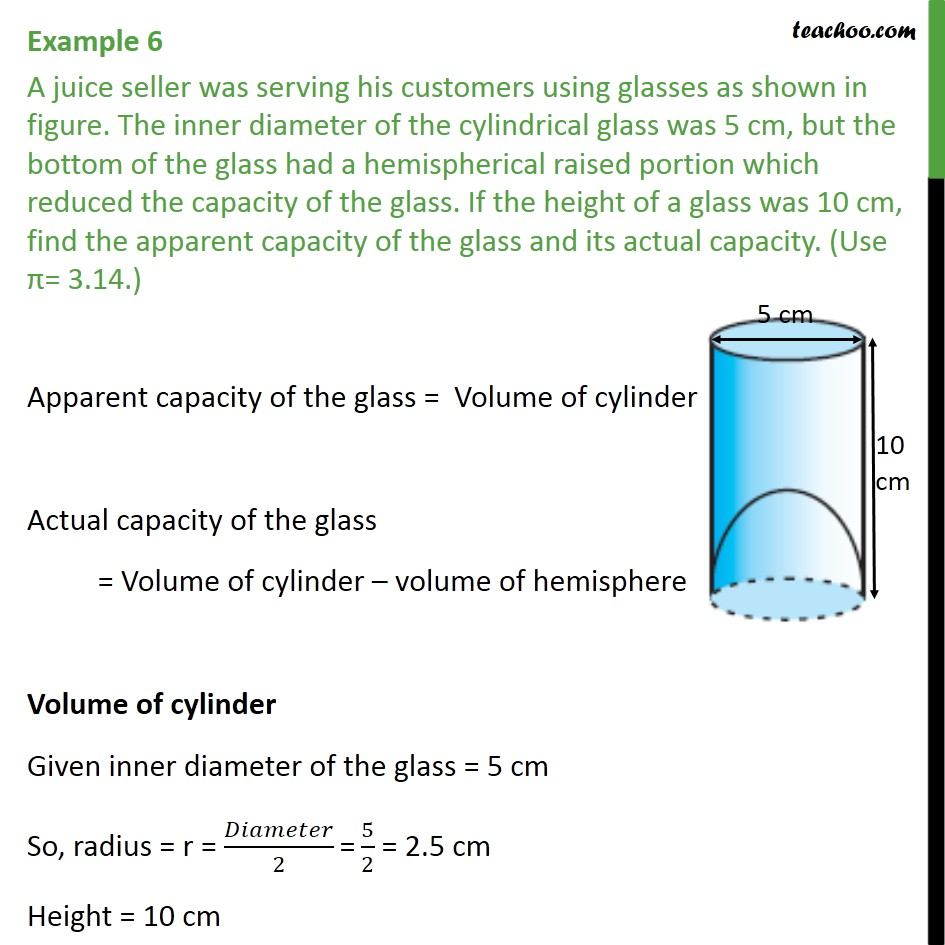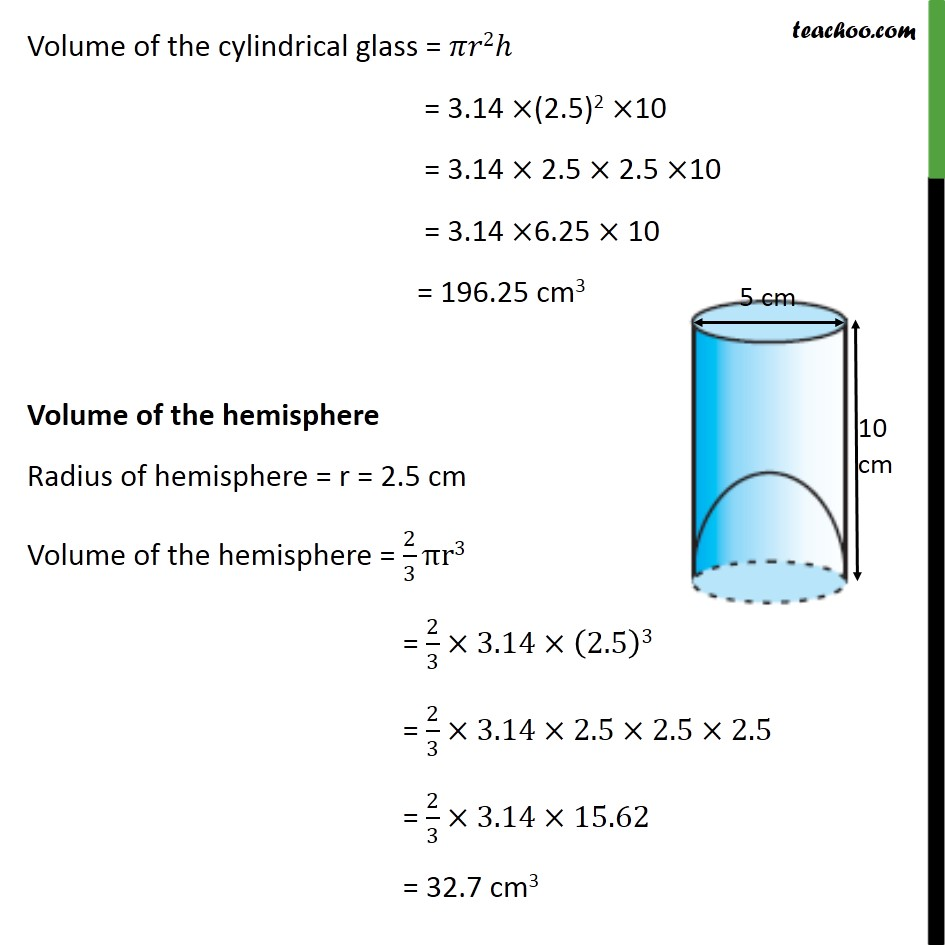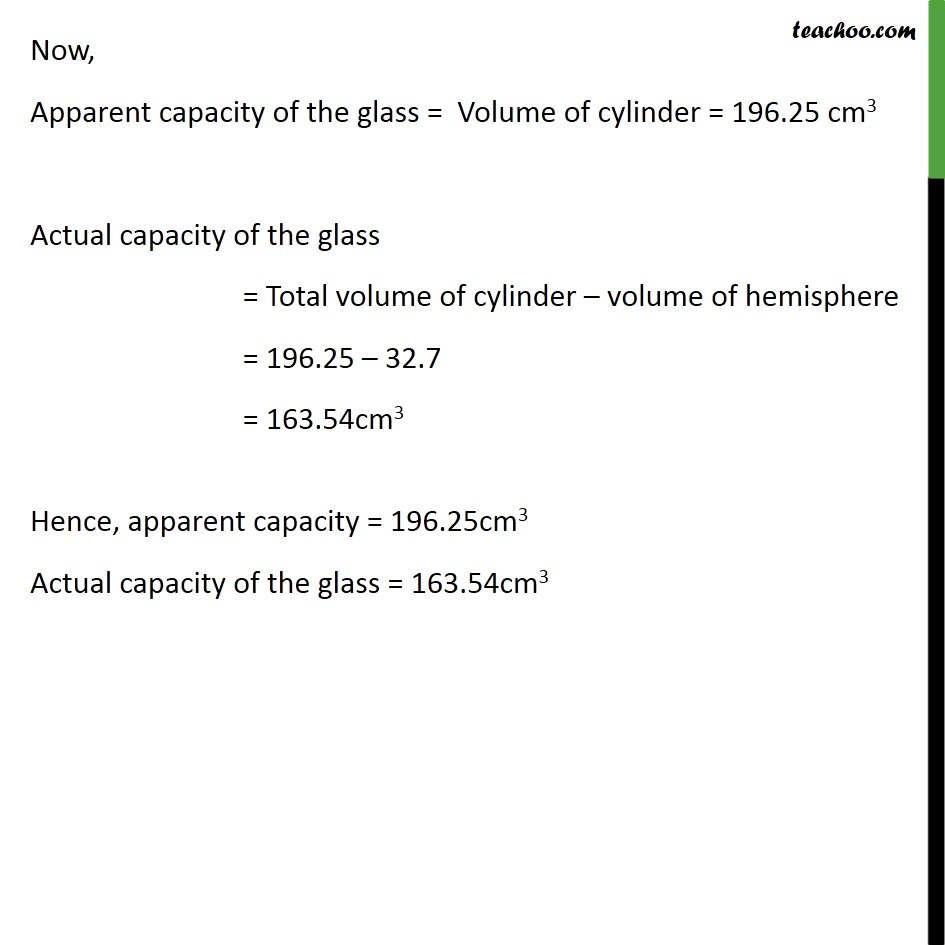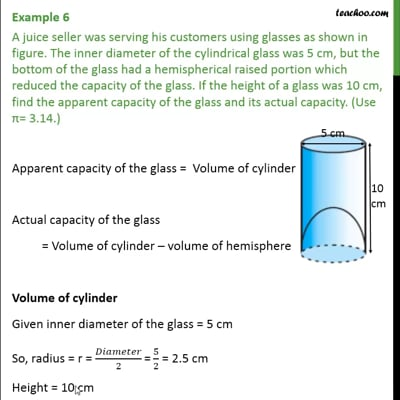Chapter 13 Class 10 Surface Areas and Volumes

Class 10
Important Questions for Exam - Class 10This video is only available for Teachoo black users

Introducing your new favourite teacher - Teachoo Black, at only ₹83 per month

### Transcript

Example 6 A juice seller was serving his customers using glasses as shown in figure. The inner diameter of the cylindrical glass was 5 cm, but the bottom of the glass had a hemispherical raised portion which reduced the capacity of the glass. If the height of a glass was 10 cm, find the apparent capacity of the glass and its actual capacity. (Use = 3.14.) Apparent capacity of the glass = Volume of cylinder Actual capacity of the glass = Volume of cylinder volume of hemisphere Volume of cylinder Given inner diameter of the glass = 5 cm So, radius = r = /2 "=" 5/2 = 2.5 cm Height = 10 cm Volume of the cylindrical glass = 2 = 3.14 (2.5)2 10 = 3.14 2.5 2.5 10 = 3.14 6.25 10 = 196.25 cm3 Volume of the hemisphere Radius of hemisphere = r = 2.5 cm Volume of the hemisphere = 2/3 r3 = 2/3 3.14 (2.5)3 = 2/3 3.14 2.5 2.5 2.5 = 2/3 3.14 15.62 = 32.7 cm3 Now, Apparent capacity of the glass = Volume of cylinder = 196.25 cm3 Actual capacity of the glass = Total volume of cylinder volume of hemisphere = 196.25 32.7 = 163.54cm3 Hence, apparent capacity = 196.25cm3 Actual capacity of the glass = 163.54cm3